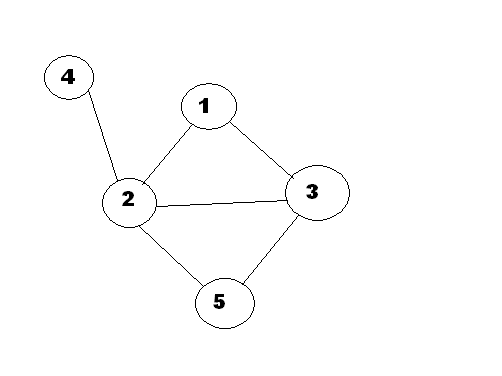GabrielxD

• 累计撰写 673 篇文章
• 累计创建 128 个标签
• 累计收到 15 条评论

### 目 录CONTENT# 【DFS】网络寻路【蓝桥杯】2023-04-24 / 0 评论 / 0 点赞 / 86 阅读 / 962 字

## 题目

X 国的一个网络使用若干条线路连接若干个节点。节点间的通信是双向的。某重要数据包，为了安全起见，必须恰好被转发两次到达目的地。该包可能在任意一个节点产生，我们需要知道该网络中一共有多少种不同的转发路径。1 -> 2 -> 3 -> 1 是允许的; 1 -> 2 -> 1-> 2 或者 1->2->3->2 都是非法的。

3 3
1 2
2 3
1 3


6


• 最大运行时间：1s
• 最大运行内存: 256M

## 解题

### 方法一：DFS

#### 思路

$1\dots n$ 每个点为起点尝试进行 DFS ，找到共走 $4$ 个节点且不经过重复节点（终点可以与起点重复）的合法路径即可增加答案。

$dfs(u, cnt, src)$$u$ 表示当前节点， $cnt$ 表示当前路径经过了多少个点， $src$ 表示当前路径的起点 ）：

• 出口：当 $cnt = 4$ 时，说明当前路径是经过了 $4$ 个点的合法路径，增加答案，退出递归；
• 枚举与点 $u$ 相连的顶点 $v$ 尝试作为路径上的第 $cnt + 1$ 个顶点：
• 如果当前不是在枚举终点（ $cnt \neq 3$ ）且枚举到了起点（ $v = src$ ），说明该顶点不能作路径上的第 $cnt + 1$ 个点，跳过本次迭代；
• 如果没有枚举到路径上已有到点（ $vis[v] = false$ ），或是当前在枚举终点且枚举到了起点（ $cnt = 3, v = src$ ），尝试把 $v$ 作为路径上的第 $cnt + 1$ 个点（ $vis[v] = true$ ），递归下一个点： $dfs(v, cnt + 1, src)$
• 回溯（ $vis[v] = false$ ）。

#### 代码

import java.util.*;
import java.io.*;

public class Main {
static int n, m;
static int[] h, e, ne;
static int idx;
static boolean[] vis;
static int ans;

static void add(int a, int b) {
e[idx] = b;
ne[idx] = h[a];
h[a] = idx++;
}

static void dfs(int u, int cnt, int src) {
if (cnt == 4) {
++ans;
return;
}
for (int i = h[u]; i != -1; i = ne[i]) {
int v = e[i];
if (cnt != 3 && v == src) continue;
if (!vis[v] || (cnt == 3 && v == src)) {
vis[v] = true;
dfs(v, cnt + 1, src);
vis[v] = false;
}
}
}

public static void main(String[] args) throws IOException {
in.nextToken(); n = (int) in.nval;
in.nextToken(); m = (int) in.nval;
h = new int[n + 1];
Arrays.fill(h, -1);
e = new int[m * 2];
ne = new int[m * 2];
while (m-- > 0) {
in.nextToken(); int u = (int) in.nval;
in.nextToken(); int v = (int) in.nval;